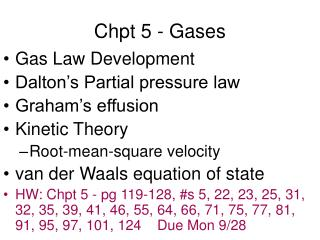DownloadDownload PresentationChpt 5 - Gases

# Chpt 5 - Gases

Download Presentation## Chpt 5 - Gases

- - - - - - - - - - - - - - - - - - - - - - - - - - - E N D - - - - - - - - - - - - - - - - - - - - - - - - - - -
##### Presentation Transcript

1. Chpt 5 - Gases • Gas Law Development • Dalton’s Partial pressure law • Graham’s effusion • Kinetic Theory • Root-mean-square velocity • van der Waals equation of state • HW: Chpt 5 - pg 119-128, #s 5, 22, 23, 25, 31, 32, 35, 39, 41, 46, 55, 64, 66, 71, 75, 77, 81, 91, 95, 97, 101, 124 Due Mon 9/28

2. Torricelli barometer Pressure is? Units? The height in mm of mercury above the surface of the resevoir of mercury determines the pressure. The units are mmHg. mmHg is also the same unit as Torr. i.e. standard pressure is 760 mmHg and 760 Torr

3. Simple Manometer Similar to the barometer, the height difference of the Hg relates the pressure difference in the unknown gas bulb side to the current atmospheric pressure. The higher Hg side has the _____ pressure. (higher/lower)

4. Boyle’s Law • Constant temperature experiments demonstrated the PV=constant graphing this yields an inverse relationship • Thus if the pressure of volume changes at a constant temperature P1V1 = P2V2

5. Charles’s Law • Constant pressure experiments demonstrated that Volume is directly proportional to Temperature (Kelvin) V1 = V2 T1 T2 • Several gases were used & all extrapolate to zero volume and the same temperature at negative 273oC

6. Plots of V vs. T(ºC) Charles’s Law Experiment results

7. Combined Gas Law P1V1 = P2V2 T1 T2 Avogadro’s Law - equal volumes of gas contain equal particles of gas V = k n At constant temperature and pressure the volume is directly proportional to the number of moles of gas.

8. Ideal Gas Law • Putting it all together, we can calculate that constant now. The universal gas constant R. PV=R or PV=nRT nT R =0.0821 l*atm/mol*K =8.31 l*kpa/mol*K

9. Effusion of Gas into Evacuated Chamber If more than one type of gas or more than one isotope, which gas effuses faster? Lighter gas moves Faster!! KE = 1/2 mv2

10. Relative Molecular Speed Distribution of H2 and UF6

11. Diffusion Rates of NH3and HCl Molecules Through Air

12. Plots of PV/nRT vs. P for Several Gases

13. Plot of PV/nRT vs. P for N2 Gas

14. Plot of PV vs. P for Several Gases

15. Values of the van der Waals Constants for Common Gases